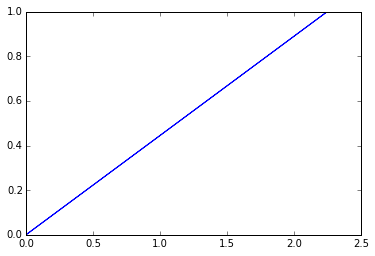# Chapter5-Introducton to Differential Analysis of Fluid Motion¶

## Ex2-pg188¶

In :
##Distance f piston from closed end of the cylinder at the give instant(in m):
#calculate Rate of change of density with time
L=0.15;
##Density of gas(in kg/m**3):
d=18.;
##Velocity of piston(in m/sec):
V=12.;
##Rate of change##
##Rate of change of density with time(in kg/m**3-s):
r=-d*V/L
print("RESULTS")
print'%s %.2f %s'%("Rate of change of density with time: ",r," kg/m^3-s")

RESULTS
Rate of change of density with time:  -1440.00  kg/m^3-s


## Ex7-pg209¶

In :
import math
#calculate "Rate of angular deformation and Rate of rotation
#Value of (in mm/sec):
U=4.;
##Value of h(in mm):
h=4.;
##Tme at which to find position(in sec):
t=1.5;

##angular and rotation##
##At point b, u=3 mm/sec
u=3.;
##Displacemet of b(in mm):
xb=u*t
##Rate of angular deformation(in s**-1):
D=U/h
##Rate of rotation(in s**-1):
rot=-0.5*U/h
print("RSULTS")
print'%s %.2f %s'%("Rate of angular deformation:",D," /sec")
print'%s %.2f %s'%("Rate of rotation: ",rot," /sec")

RSULTS
Rate of angular deformation: 1.00  /sec
Rate of rotation:  -0.50  /sec


## Ex8-pg 210¶

In :
##Value of A(in sec**-1):
#calculate Rates of linear deformation in X and Y direction:  Rate of volume dilation
A=0.3;
import numpy
import math
import matplotlib
from matplotlib import pyplot
%matplotlib inline
import warnings
from math import log
warnings.filterwarnings('ignore')
##Rates and area##

##Value of T:
T=math.log(3./2.)/A
x0=numpy.linspace(1,2,num=3);
i=len(x0)
X=numpy.zeros(i)

for i in range (1,2):
X[i]=x0[i]*math.e**(A*T)
y0=numpy.linspace(1,2,num=3);
j=len(y0)
Y=numpy.zeros(j)
for j in range(1,2):
Y[i]=y0[j]*math.e**(-A*T)
##For X coordinate:
##For Y coordinate:

pyplot.plot(X,Y)
##Rates of linear deformation in X direction:
Ax=0.3;
##Rate of linear deformation in the y direction:
Ay=-0.3;
##Rate of volume dilation(s**-1):
v=A-A
##Area of abcd:
A1=1.;
##Area of a'b'c'd':
A2=(3.-3./2.)*(4./3.-2./3.)
print("RESULTS")
print"%s %.2f %s %.2f %s "%("Rates of linear deformation in X and Y direction: ",Ax," /s"and "",Ay," /s")
print"%s %.2f %s"%("Rate of volume dilation: ",v," /sec")
print"%s %.2f %s %.2f %s "%("Area of abcd and a,b,c,d:",A1," m^2"and" ",A2," m^2")

RESULTS
Rates of linear deformation in X and Y direction:  0.30  -0.30  /s
Rate of volume dilation:  0.00  /sec
Area of abcd and a,b,c,d: 1.00   1.00  m^2## Ex9-pg215¶

In :
import math
#Volume flow rate
##Thickness of water film(in mm):
h=1.;
##Width of surface(in m):
b=1.;
##Angle of inclination of surface:
theta=15.;
##Density of water(in kg/m**3):
d=999.;
##Acceleration du to gravity(in m/sec**2):
g=9.81;
##Viscosity(kg/m-s):
u=10**-3.;##Volume flow rate##
##Volume flow rate(in m**3/sec):
Q=d*g*math.sin(theta/57.3)*b*(h/1000.)**3.*1000./u/3.
print("RESULTS")
print'%s %.2f %s'%("Volume flow rate: ",Q," m^3/sec")

RESULTS
Volume flow rate:  0.85  m^3/sec